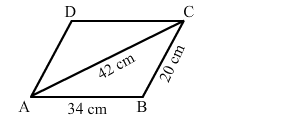# The adjacent sides of a ∥gm ABCD measure 34 cm and 20 cm and the diagonal AC is 42 cm long.`
Question:

The adjacent sides of a ∥gm ABCD measure 34 cm and 20 cm and the diagonal AC is 42 cm long. Find the area of the ∥gm.Solution:

The diagonal of a parallelogram divides it into two congruent triangles. Also, the area of the parallelogram is the sum of the areas of the triangles.

We will now use Hero's formula to calculate the area of triangle ABC.

Semiperimeter, $s=\frac{1}{2}(34+20+42)=\frac{1}{2}(96)=48 \mathrm{~cm}$

Area of $\Delta \mathrm{ABC}=\sqrt{s(s-a)(s-b)(s-c)}$

$=\sqrt{48(48-42)(48-34)(48-20)}$

$=\sqrt{48 \times 6 \times 14 \times 28}$

$=\sqrt{112896}$

$=336 \mathrm{~cm}^{2}$

Area of the parallelogram $=2 \times$ Area $\Delta \mathrm{ABC}=2 \times 336=672 \mathrm{~cm}^{2}$